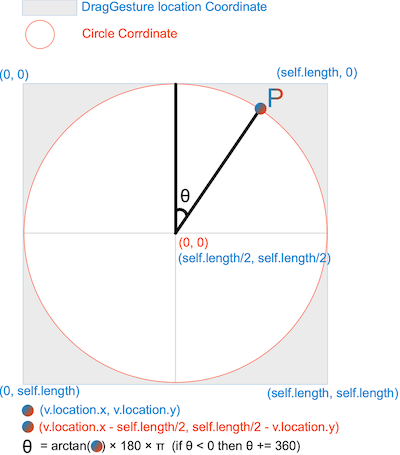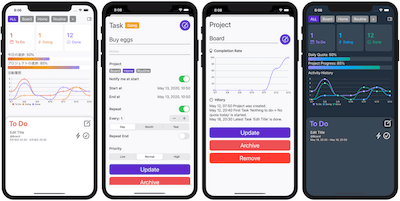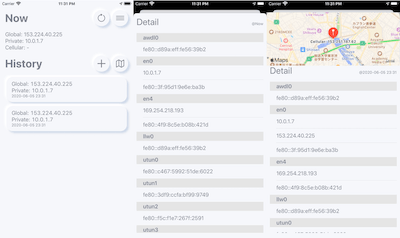# Rotate with DragGesture

`DragGesture` can only get the coordinates of the moved position or distance. how to get the rotation angle and how to implement it, which is necessary to handle the rotation using atan2.

## Calculate the angle of rotation

You need to understand the how to calculate the rotation angle before implementing the rotation in `DragGesture`In `DragGesture`, you need to calculate the rotation angle yourself.

The figure shows the idea needed to find the rotation from which can be obtained with `location:CGPoint` from `DragGesture`.

Blue is the coordinate system of `DragGesture`, and orange is the coordinate of the rotating body It is necessary to find the rotation angle θ in the system.

the point P become `(v.location.x - self.length / 2, self.length / 2 - v.location.y)` with them and the diameter of the rotating body `self.length`.

And the rotation angle is θ=arctan(v.location.x - self.length / 2 , self.length / 2 - v.location.y) * 180 * π (θ < 0 ? θ += 360).## Implementing with `DragGesture` Part 1

Rotation angle of the object = Rotation angle of the gesture

This is an implementation method where the angle is reset each time the object is rotated.
We use `atan2` (arc tangent) to find the rotation angle θ and pass it to `rotationEffect`.

``````//
//  ContentView.swift
//

import SwiftUI

struct ContentView: View {
@State private var angle: CGFloat = 0
@State private var length : CGFloat = 400

var body: some View {
Image("ice")
.resizable()
.aspectRatio(contentMode: .fill)
.clipShape(Circle())
.frame(width: length, height: length)
.rotationEffect(.degrees(Double(self.angle)))
.gesture(DragGesture()
.onChanged{ v in
self.angle = atan2(v.location.x - self.length / 2, self.length / 2 - v.location.y) * 180 / .pi
if (self.angle < 0) { self.angle += 360 }
}
)
}
}

struct ContentView_Previews: PreviewProvider {
static var previews: some View {
ContentView()
}
}``````

## Implementing with `DragGesture` Part 2

Rotation angle of the object += Rotation angle of the gesture

I'll also show you how to implement it by adding a rotation angle each time you rotate the object.
`atan2` (arc-tangent) for the starting point to find the difference between the rotation angle and the rotation angle θ of the point being moved.
When the rotation is complete, save the final angle in `lastAngle` and add it to the rotation angle difference for the next rotation.

``````//
//  ContentView.swift
//

import SwiftUI

struct ContentView: View {
@State private var angle: CGFloat = 0
@State private var lastAngle: CGFloat = 0
@State private var length : CGFloat = 400

var body: some View {
Image("ice")
.resizable()
.aspectRatio(contentMode: .fill)
.clipShape(Circle())
.frame(width: length, height: length)
.rotationEffect(.degrees(Double(self.angle)))
.gesture(DragGesture()
.onChanged{ v in
var theta = (atan2(v.location.x - self.length / 2, self.length / 2 - v.location.y) - atan2(v.startLocation.x - self.length / 2, self.length / 2 - v.startLocation.y)) * 180 / .pi
if (theta < 0) { theta += 360 }
self.angle = theta + self.lastAngle
}
.onEnded { v in
self.lastAngle = self.angle
}
)
}
}

struct ContentView_Previews: PreviewProvider {
static var previews: some View {
ContentView()
}
}``````
Gestures | Apple Developer Documentation
geometry - calculating angle between two points on edge of circle Swift SpriteKit - Stack Overflow

### ToDo App

Visualize Activity, Data sharing with iCloud, Dark mode supported.